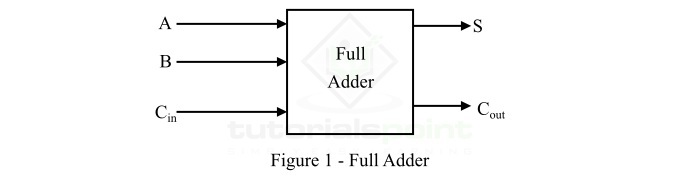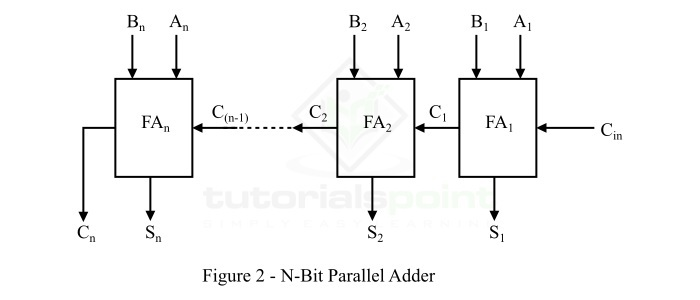Let us stat this article with a brief introduction of binary adders and rules of binary addition. In digital electronics, an adder or binary adder is a combinational digital circuit which performs the addition of two or more binary digits. The binary addition of two bits is performed by following these four rules −

            0 + 0 = 0
0 + 1 = 1
1 + 0 = 1
1 + 1 = 10 (Sum = 0; Carry = 1)


The first three operations produce a sum whose bit length is one binary digit. But, the sum of last combination, i.e. when augend and addend both are equal to 1, the binary sum consists of two binary digits namely, sum bit and carry bit. The most significant bit is the carry bit, while the least significant bit is the sum bit.

We also require the knowledge of full-adder circuit for better understanding of the implementation and operation of an N-bit parallel adder. The full-adder along with its block diagram and truth table is describe below.

A combination digital circuit that adds two bits and a carry bit and produces a sum bit and a carry bit as output is referred to as a full adder (FA).

In other words, a binary adder circuit that can add three input bits and produces two output bits, i.e. sum bit and carry bit is called full adder. The block diagram of a full adder is shown in Figure-1.Here, A and B are the input bits, Cin is the input carry bit from previous sum, S is the output sum bit, and Cout is the output carry bit.

The operation of the full adder circuit can be understood easily from its truth table which is given below.

Input Output
A B Cin S Cout
00000
00110
01010
01101
10010
10101
11001
11111

Now, let us discuss the realization of an N-bit parallel adder using full adders.

Parallel adder is a binary adder circuit implemented to add two binary number having N-bits (for example, to add 4-bit binary numbers, we use 4- bit parallel adder, and so on). As its name implies, the parallel adder is a digital combinational circuit that adds two binary numbers in parallel form and generates the arithmetic sum of those binary numbers in parallel form.

As we already mentioned above that a full adder can perform addition of only two one-bit binary numbers consisting of two input bits and one input carry bit, i.e. addition of three bits. But in actual practice, we have to add such binary numbers whose length is more than one bit. To add such binary numbers, we use parallel binary adder which is capable of adding the two binary numbers of any bit length such as 4-bit, 5-bit, etc.

We can implement an N-bit parallel adder with the help of full-adders connected in a chain fashion. The block diagram representation of an N-bit parallel adder using full adders is shown in Figure-2.From the block diagram of the N-bit parallel adder, it can be seen that the carry output from each full-adder is connected to the carry input terminal of the next higher level full-adder in the chain.

The number of full-adder to realize a parallel adder is determined from the number of bits in the two binary numbers to be added. Therefore, an N-bit parallel adder requires N full-adders to perform the addition in parallel form. For example, a 2-bit parallel adder requires 2 full adders, 4-bit parallel adder consists of 4 full adders, and so on.

## Operation of N-Bit Parallel Adder Circuit

The working of the N-bit parallel adder shown in figure-2 can be described in the following steps −

• Initially, the full adder FA1 adds two input bits A1 and B1 along with an input carry bit Cin, and it generates the output sum bit S1 and the carry bit C1 which is forwarded to the next adder (FA2) in the chain. The sum bit S1 is the least significant bit of the output sum.

• At the next stage, the full adder circuit FA2 becomes active and adds input bits A2 and B2 along with C1. It generates the sum bit S2 which is the second bit of the output sum, and the carry bit C2 that is connected to the next full adder FA3 in the chain.

• This process will continue till the last full adder, i.e. FAn in the chain. The full adder uses carry input C(n-1) to add with the input bits An and Bn to produce the last bit of the output sum Sn and the last output carry bit Cn.

• It makes addition of binary numbers fast.

• Parallel adder is more economical.

The major disadvantage of the parallel adder is propagation delay. Since, in the parallel adder, carry from previous addition has to be propagated to the next adder which takes some time. This cause a significant propagation delay in the addition. This propagation delay is directly proportional to the number of bits in the binary numbers.

The important applications of parallel adders are listed below −

• Parallel adders are used in arithmetic logic units that are used for heavy computing applications.

• Parallel adders are also used in parallel cellular automatic machines for parallel computing.

• Parallel adders are utilized for conversion of BCD into excess-1 code.

• Parallel adders are also used for the analysis of multiplication algorithms.

## Conclusion

This is all about n-bit parallel adder in digital electronics. From the above discussion, we can conclude that the n-bit parallel adder is a combination digital circuit which is implemented using n full-adders to add two binary numbers in parallel form. The parallel adder performs addition of bits simultaneously, hence it increases the speed of binary addition.

Updated on: 19-Apr-2023

2K+ Views